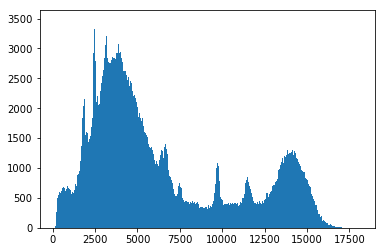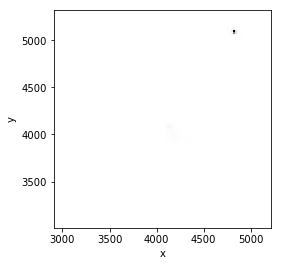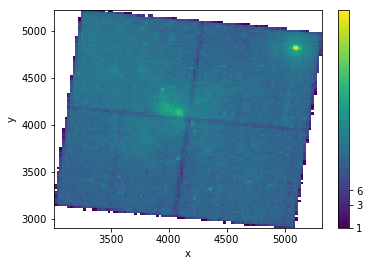In :
import numpy as np

from astropy.io import fits

import matplotlib.pyplot as plt
%matplotlib inline


The following line is needed to download the example FITS files used in this tutorial.

In :
from astropy.utils.data import download_file


# Viewing and manipulating data from FITS tables¶

In :
from astropy.io import fits


FITS files can often contain large amount of multi-dimensional data and tables.

In this particular example, I will open a FITS file from a Chandra observation of the Galactic Center. The file contains a list of events with x and y coordinates, energy, and various other pieces of information.

In :
event_filename = download_file( 'http://data.astropy.org/tutorials/FITS-tables/chandra_events.fits', cache=True )


## Opening the FITS file and viewing table contents¶

Since the file is big, I will open with memmap=True to prevent RAM storage issues.

In :
hdu_list = fits.open(event_filename, memmap=True)

In :
hdu_list.info()

Filename: /Users/erik/.astropy/cache/download/py3/26e9900d731d08997d99ada3973f4592
No.    Name         Type      Cards   Dimensions   Format
0  PRIMARY     PrimaryHDU      30   ()
1  EVENTS      BinTableHDU    890   483964R x 19C   [1D, 1I, 1I, 1J, 1I, 1I, 1I, 1I, 1E, 1E, 1E, 1E, 1J, 1J, 1E, 1J, 1I, 1I, 32X]
2  GTI         BinTableHDU     28   1R x 2C   [1D, 1D]
3  GTI         BinTableHDU     28   1R x 2C   [1D, 1D]
4  GTI         BinTableHDU     28   1R x 2C   [1D, 1D]
5  GTI         BinTableHDU     28   1R x 2C   [1D, 1D]
6  GTI         BinTableHDU     28   1R x 2C   [1D, 1D]


I'm interested in reading EVENTS, which contains information about each X-ray photon that hit the detector.

To find out what information the table contains, I will print the column names.

In :
print(hdu_list.columns)

ColDefs(
name = 'time'; format = '1D'; unit = 's'
name = 'ccd_id'; format = '1I'
name = 'node_id'; format = '1I'
name = 'expno'; format = '1J'
name = 'chipx'; format = '1I'; unit = 'pixel'
name = 'chipy'; format = '1I'; unit = 'pixel'
name = 'tdetx'; format = '1I'; unit = 'pixel'
name = 'tdety'; format = '1I'; unit = 'pixel'
name = 'detx'; format = '1E'; unit = 'pixel'
name = 'dety'; format = '1E'; unit = 'pixel'
name = 'x'; format = '1E'; unit = 'pixel'
name = 'y'; format = '1E'; unit = 'pixel'
name = 'pha'; format = '1J'; unit = 'adu'; null = 0
name = 'pha_ro'; format = '1J'; unit = 'adu'; null = 0
name = 'energy'; format = '1E'; unit = 'eV'
name = 'pi'; format = '1J'; unit = 'chan'; null = 0
name = 'fltgrade'; format = '1I'
name = 'grade'; format = '1I'
name = 'status'; format = '32X'
)


Now I'll we'll take this data and convert it into an astropy table. While it is possible to access FITS tables directly from the .data attribute, using Table tends to make a variety of common tasks more convenient.

In :
from astropy.table import Table

evt_data = Table(hdu_list.data)


For example, a preview of the table is easily viewed by simply running a cell with the table as the last line:

In :
evt_data

Out:
<Table length=483964>
float64int16int16int32int16int16int16int16float32float32float32float32int32int32float32int32int16int16bool
238623220.90933689208512439815095.644139.04168.075087.773548353413874.7951164False .. False
238623220.9093168437237489534984865.574621.183662.24915.936676292621.19180642False .. False
238623220.9093268719289484337804814.834340.253935.224832.553033287512119.083183False .. False
238623220.9093068103295483731644807.364954.383324.464897.288317733253.0422300False .. False
238623220.9093168498314481835594788.994560.333713.634832.733612343914214.4974642False .. False
238623220.9093368791469466338524635.454268.053985.854645.935004381952.7213400False .. False
238623220.9093368894839429339554266.644165.324044.554267.68357133267.5322400False .. False
238623220.9093368857941419139184164.814202.233995.944170.829758043817.0426200False .. False
238623220.9093368910959417339714146.994149.364046.344146.915764462252.7315500False .. False
238623220.9093368961962417040224144.134098.54096.524138.09157213546154.1142200False .. False
.........................................................
238672393.551315723933199493350404902.913082.55212.54766.23122211814819.8333100False .. False
238672393.551215723596412472047034691.513418.994853.514595.83142302012536.9859106False .. False
238672393.5513157231000608452451074494.713015.725230.894353.026585852599.5717900False .. False
238672393.551115723270917421543774188.333743.64472.074134.223861346315535.81024164False .. False
238672393.551015723232988414443394117.613781.884425.754068.49168014996653.0845600False .. False
238672393.5910115723366103316447663140.93356.324733.683048.573621360214362.598400False .. False
238672393.5910315723937646370741953681.213925.554231.843651.973717348614654.0100483False .. False
238672393.5910115723406687374847263723.43396.254762.423631.72167615366652.8345600False .. False
238672393.5910115723354870393147783906.073344.774834.993807.08243621659672.88663164False .. False
238672393.6326115723384821325925233230.925596.852519.223401.034913561875.9412900False .. False

We can extract data from the table by referencing the column name.. For example, I'll make a histogram for the energy of each photon, giving us a sense for the spectrum (folded with the detector's efficiency).

In :
NBINS = 500
energy_hist = plt.hist(evt_data['energy'], NBINS)## Making a 2-D histogram with some table data¶

I will make an image by binning the x and y coordinates of the events into a 2-D histogram.

This particular observation spans five CCD chips. First we determine the events that only fell on the main (ACIS-I) chips, which have number ids 0, 1, 2, and 3.

In :
ii = np.in1d(evt_data['ccd_id'], [0, 1, 2, 3])
np.sum(ii)

Out:
434858

### Method 1: Use numpy to make a 2-D histogram and imshow to display it¶

This method allowed me to create an image without stretching

In :
NBINS = (100,100)

img_zero, yedges, xedges = np.histogram2d(evt_data['x'][ii], evt_data['y'][ii], NBINS)

extent = [xedges, xedges[-1], yedges, yedges[-1]]

plt.imshow(img_zero, extent=extent, interpolation='nearest', cmap='gist_yarg', origin='lower')

plt.xlabel('x')
plt.ylabel('y')

# To see more color maps
# http://wiki.scipy.org/Cookbook/Matplotlib/Show_colormaps

Out:
<matplotlib.text.Text at 0x117503d68>### Method 2: Use hist2d with a log-normal color scheme¶

In :
from matplotlib.colors import LogNorm

In :
NBINS = (100,100)
img_zero_mpl = plt.hist2d(evt_data['x'][ii], evt_data['y'][ii], NBINS, cmap='viridis', norm=LogNorm())

cbar = plt.colorbar(ticks=[1.0,3.0,6.0])
cbar.ax.set_yticklabels(['1','3','6'])

plt.xlabel('x')
plt.ylabel('y')

Out:
<matplotlib.text.Text at 0x117de1198>## Close the FITS file¶

When you're done using a FITS file, it's often a good idea to close it. That way you can be sure it won't continue using up excess memory or file handles on your computer. (This happens automatically when you close Python, but you never know how long that might be...)

In :
hdu_list.close()


## Exercises¶

Make a scatter plot of the same data you histogrammed above. The plt.scatter function is your friend for this. What are the pros and cons of doing this?

In :



Try the same with the plt.hexbin plotting function. Which do you think looks better for this kind of data?

In :



Choose an energy range to make a slice of the FITS table, then plot it. How does the image change with different energy ranges?

In :